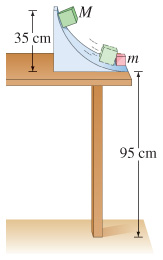Problem: In a physics lab, a cube slides down a frictionless incline as shown in the figure and elastically strikes another cube at the bottom that is only 1/4 its mass. If the incline is 35 cm high and the table is 95 cm off the floor, where does each cube land? [Hint: Both leave the incline moving horizontally.]

86% (15 ratings)
Problem Details

In a physics lab, a cube slides down a frictionless incline as shown in the figure and elastically strikes another cube at the bottom that is only 1/4 its mass. If the incline is 35 cm high and the table is 95 cm off the floor, where does each cube land? [Hint: Both leave the incline moving horizontally.]